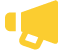Some test text!

Search PDF files for text in Swift

Sample Swift code for using PDFTron SDK to search text on PDF pages using regular expressions. The TextSearch utility class builds on functionality available in TextExtractor to simplify most common search operations. Learn more about our PDF Indexed Search Library.

To run this sample, get started with a free trial of PDFTron SDK.

//---------------------------------------------------------------------------------------
// Consult legal.txt regarding legal and license information.
//---------------------------------------------------------------------------------------

import PDFNet
import Foundation

// This sample illustrates the basic text search capabilities of PDFNet.

func runTextSearchTest() -> Int {
return autoreleasepool {
var ret: Int = 0

let input_path: String? = Bundle.main.path(forResource: "credit card numbers", ofType: "pdf")

do {
try PTPDFNet.catchException {
let doc: PTPDFDoc! = PTPDFDoc(filepath: input_path)
doc.initSecurityHandler()

let txt_search: PTTextSearch! = PTTextSearch()
var mode = e_ptwhole_word.rawValue | e_ptpage_stop.rawValue
var pattern = "joHn sMiTh"

//call Begin() method to initialize the text search.
txt_search.begin(doc, pattern: pattern, mode: mode, start_page: -1, end_page: -1)

var step: Int = 0

//call run() method iteratively to find all matching instances.
while true {
let result: PTSearchResult! = txt_search.run()

if (result != nil) {
if step == 0 {
//step 0: found "John Smith"
//note that, here, 'ambient_string' and 'hlts' are not written to,
//as 'e_ambient_string' and 'e_highlight' are not set.

print("\(result.getMatch()!)'s credit card number is: ")
//now switch to using regular expressions to find John's credit card number
mode = txt_search.getMode()
mode |= e_ptreg_expression.rawValue | e_pthighlight.rawValue
txt_search.setMode(mode)
pattern = "\\d{4}-\\d{4}-\\d{4}-\\d{4}"
//or "(\\d{4}-){3}\\d{4}"
txt_search.setPattern(pattern)

step += 1
} else if step == 1 {
//step 1: found John's credit card number
print("  \(result.getMatch()!)")

//note that, here, 'hlts' is written to, as 'e_highlight' has been set.
//output the highlight info of the credit card number.
let hlts: PTHighlights = result.getHighlights()
hlts.begin(doc)
while hlts.hasNext() {
print("The current highlight is from page: \(hlts.getCurrentPageNumber())")
hlts.next()
}

//see if there is an AMEX card number
pattern = "\\d{4}-\\d{6}-\\d{5}"
txt_search.setPattern(pattern)

step += 1
} else if step == 2 {
//found an AMEX card number
print("There is an AMEX card number: \(result.getMatch()!)")

//change mode to find the owner of the credit card; supposedly, the owner's
//name proceeds the number
mode = txt_search.getMode()
mode |= e_ptsearch_up.rawValue
txt_search.setMode(mode)
pattern = "[A-z]++ [A-z]++"
txt_search.setPattern(pattern)
step += 1
} else if step == 3 {
//found the owner's name of the AMEX card
print("Is the owner's name: \(result.getMatch()!)?")

//add a link annotation based on the location of the found instance
let hlts: PTHighlights = result.getHighlights()
hlts.begin(doc)
while hlts.hasNext() {
let cur_page: PTPage = doc.getPage(UInt32(hlts.getCurrentPageNumber()))
var i: Int = 0

while i < quads.size() {
//assume each quad is an axis-aligned rectangle
let x1: Double = min(min(min(q.getP1().getX(), q.getP2().getX()), q.getP3().getX()), q.getP4().getX())
let x2: Double = max(max(max(q.getP1().getX(), q.getP2().getX()), q.getP3().getX()), q.getP4().getX())
let y1: Double = min(min(min(q.getP1().getY(), q.getP2().getY()), q.getP3().getY()), q.getP4().getY())
let y2: Double = max(max(max(q.getP1().getY(), q.getP2().getY()), q.getP3().getY()), q.getP4().getY())
let rect = PTPDFRect(x1: x1, y1: y1, x2: x2, y2: y2)
let action = PTAction.createURI(doc.getSDFDoc(), uri: "http://www.pdftron.com")
let hyper_link = PTLink.create(withAction: doc.getSDFDoc(), pos: rect, action: action)
i += 1
}
hlts.next()
}
doc.save(toFile: URL(fileURLWithPath: NSSearchPathForDirectoriesInDomains(.documentDirectory, .userDomainMask, true)).appendingPathComponent("credit card numbers_linked.pdf").path, flags: e_ptlinearized.rawValue)

break
}
} else if (result.isPageEnd()) {
//you can update your UI here if needed
} else {
break
}
}
}
} catch let e as NSError {
print("\(e)")
ret = 1
}
return ret
}
}
close

Free Trial

Get unlimited trial usage of PDFTron SDK to bring accurate, reliable, and fast document processing capabilities to any application or workflow.

Select a platform to get started with your free trial.

Web

Android

iOS

Windows

Linux

Unlimited usage. No email address required.PDFTron Receives USD\$71 Million Growth Investment Led By Silversmith Capital Partners